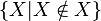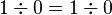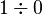# How Rodin Deviates from Abrial's Book

This page summarizes (some) differences between the version of Event-B described in Abrial's book and the version implemented by Rodin.

### Types

One may understand from Chapter 9 that Event-B is based on naive set theory, and therefore has terms like$\{X | X \notin X\}$. This is not the case in Rodin: in Rodin terms are typed similarly as in higher order logic, and$\{X | X \notin X\}$ is therefore ill-typed. For the details see the page about the Event-B Mathematical Language.

### Well-Definedness

On the one hand, the proof calculus devised in Chapter 9 allows one to prove$1\div 0 = 1\div0$. On the other hand, it is impossible to prove$1\div0=1\div0$ in Rodin, because$1\div0$ is regarded as ill-defined. Well-definedness is described here and here.

### Proof Calculus

The proof calculus used in Abrial's book is different from the proof calculus implemented by Rodin, i.e., rules have different names, and some rules available in Abrial's book are unavailable in Rodin and vice versa.